# RS Aggarwal Solutions for Class 8 Maths Chapter 25 - Probability

Download pdf of RS Aggarwal Solutions for Class 8 Maths Chapter 25- Probability, from the links given below. The solutions are created by subject matter experts based on the latest CBSE syllabus for
Class 8 which help students excel in their exams without any difficulty. With the help of different exercise questions, one can gain a better understanding of the different concepts covered in the
syllabus. And also help students understand the methods used in solving tricky problems and master the subject. Here is the RS Aggarwal Solutions for Class 8 Maths Chapter 25 where the questions are solved in detail.

## Download PDF of RS Aggarwal Solutions for Class 8 Maths Chapter 25 – Probability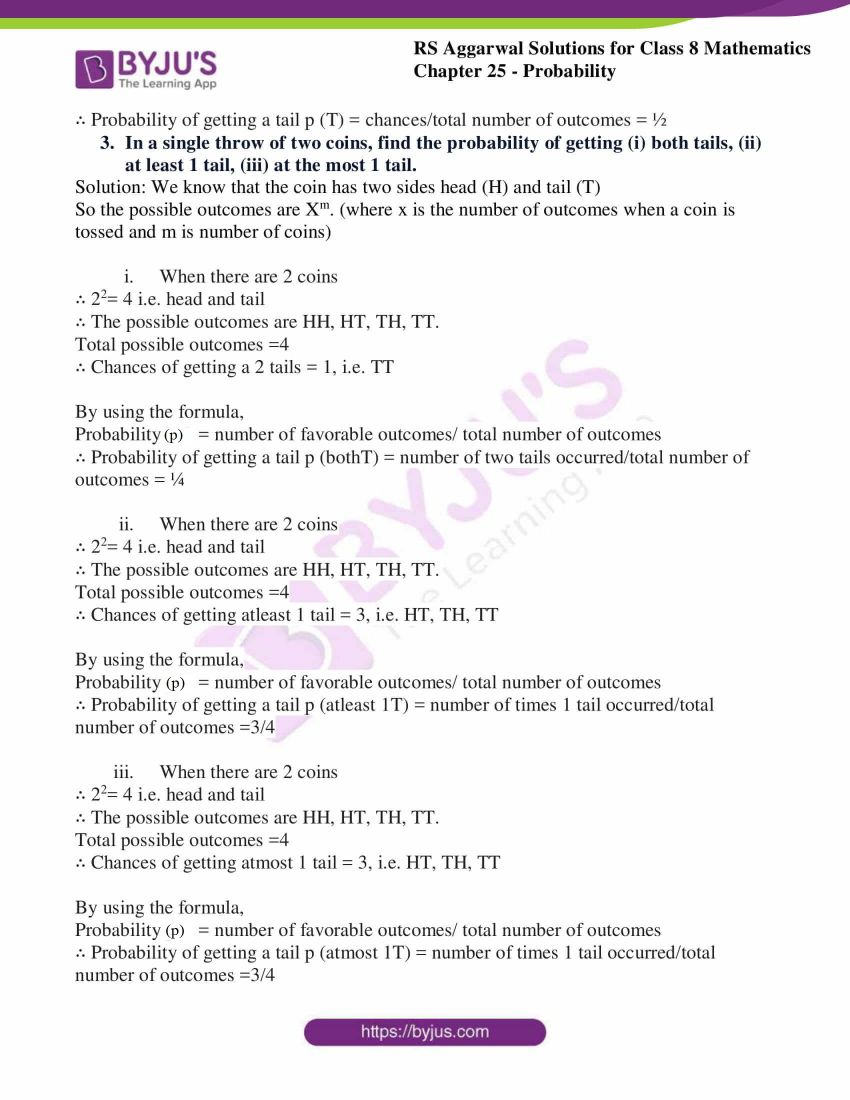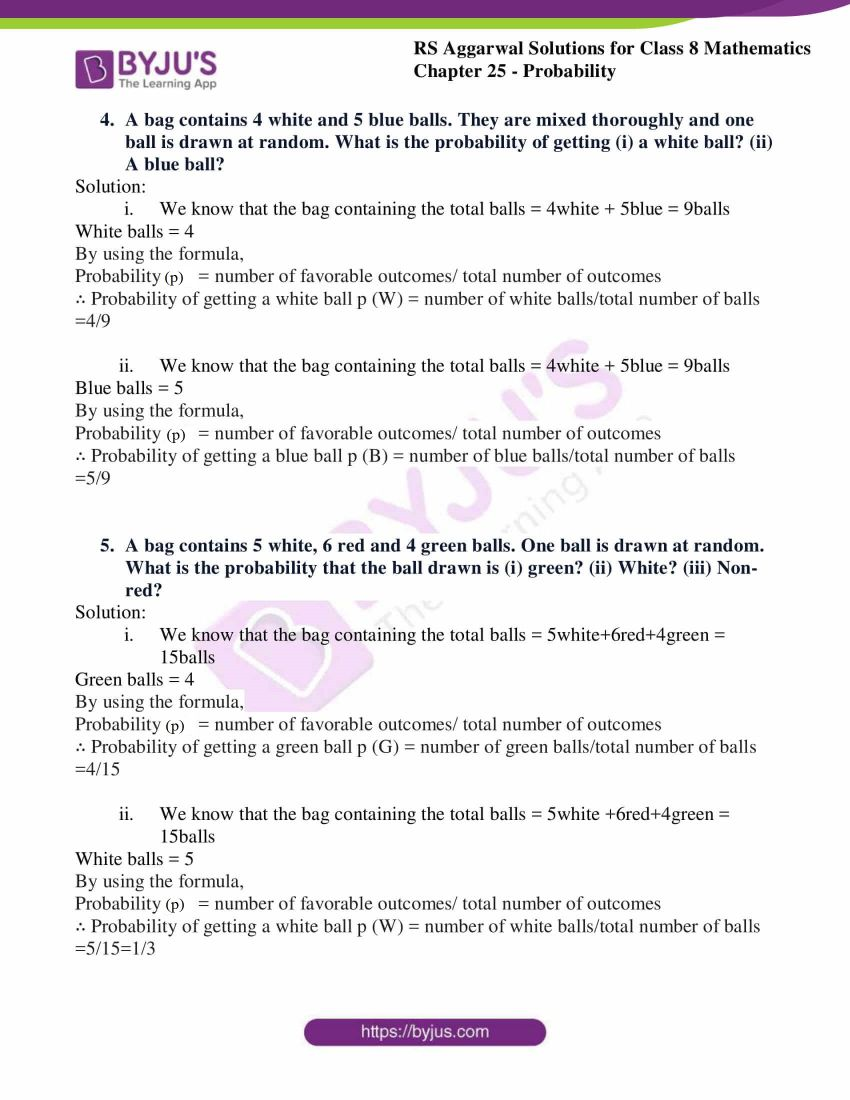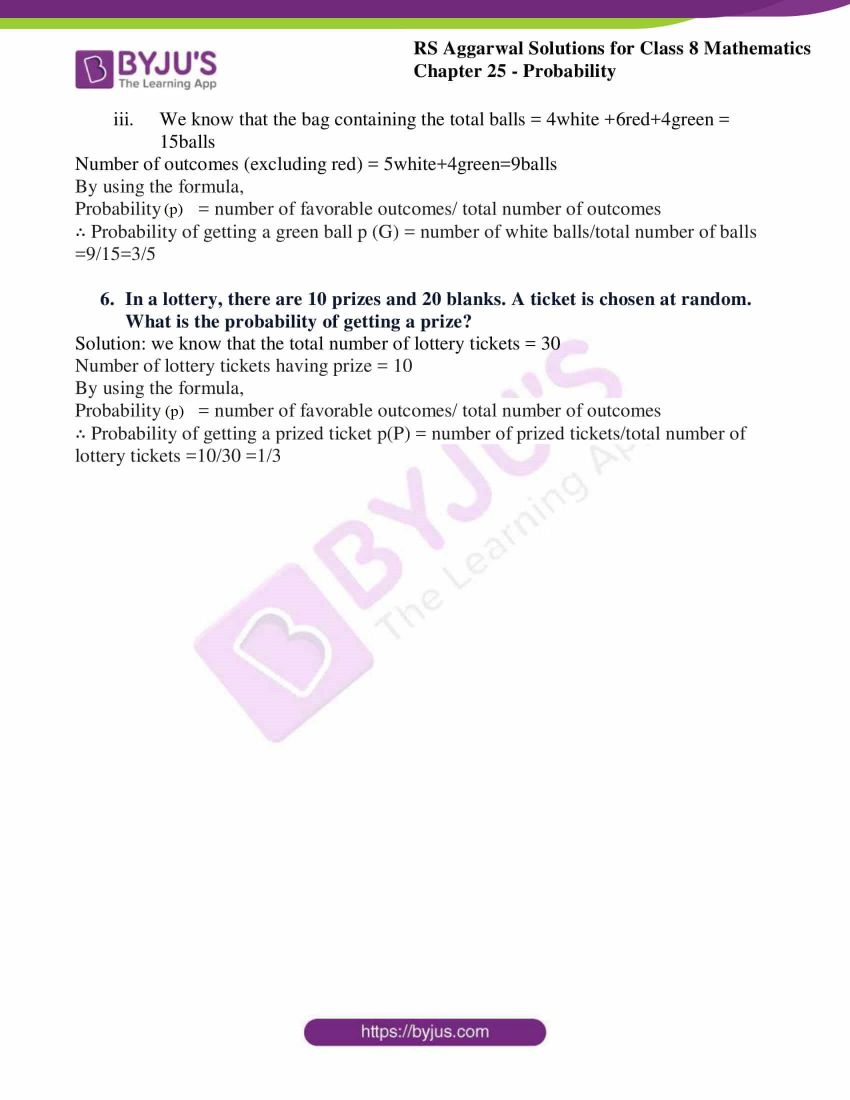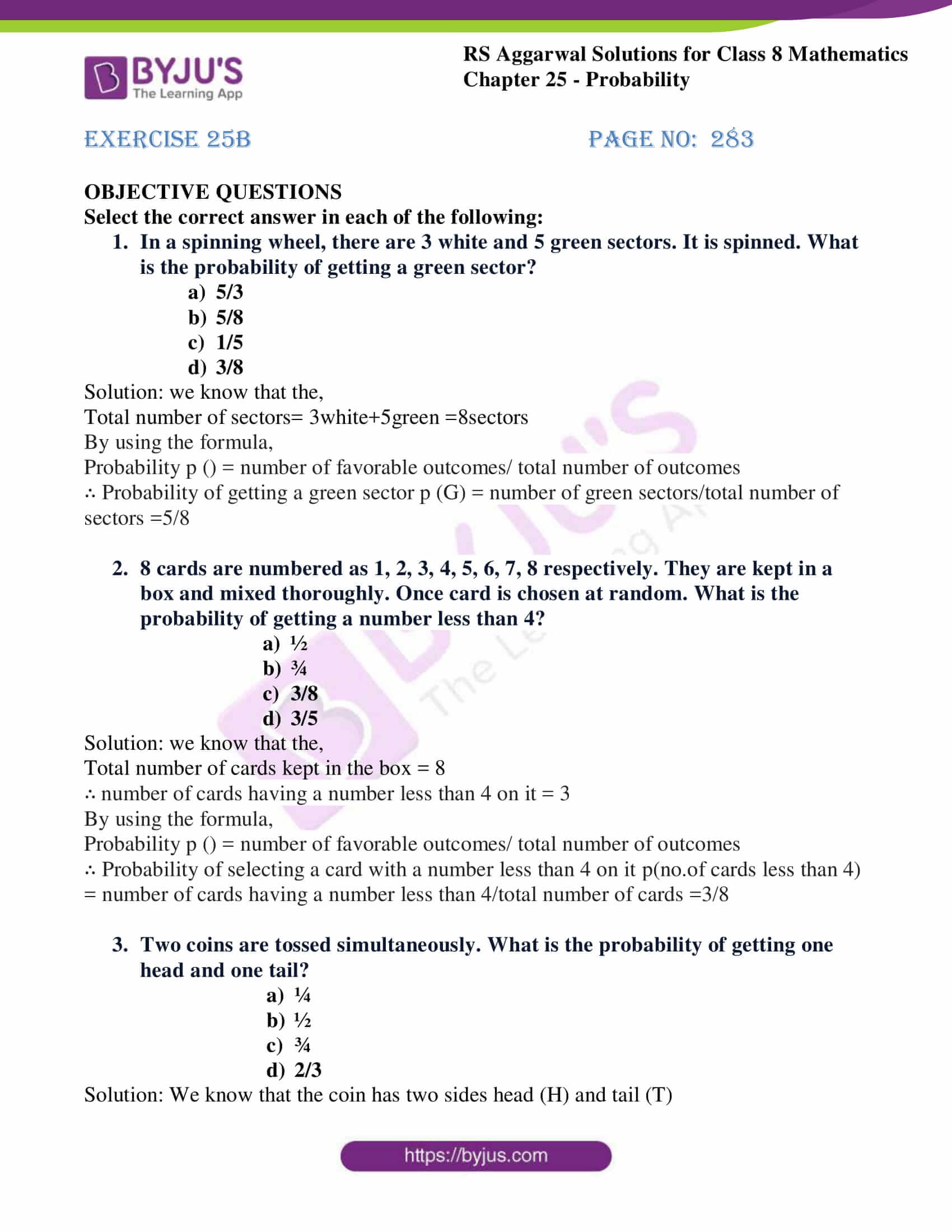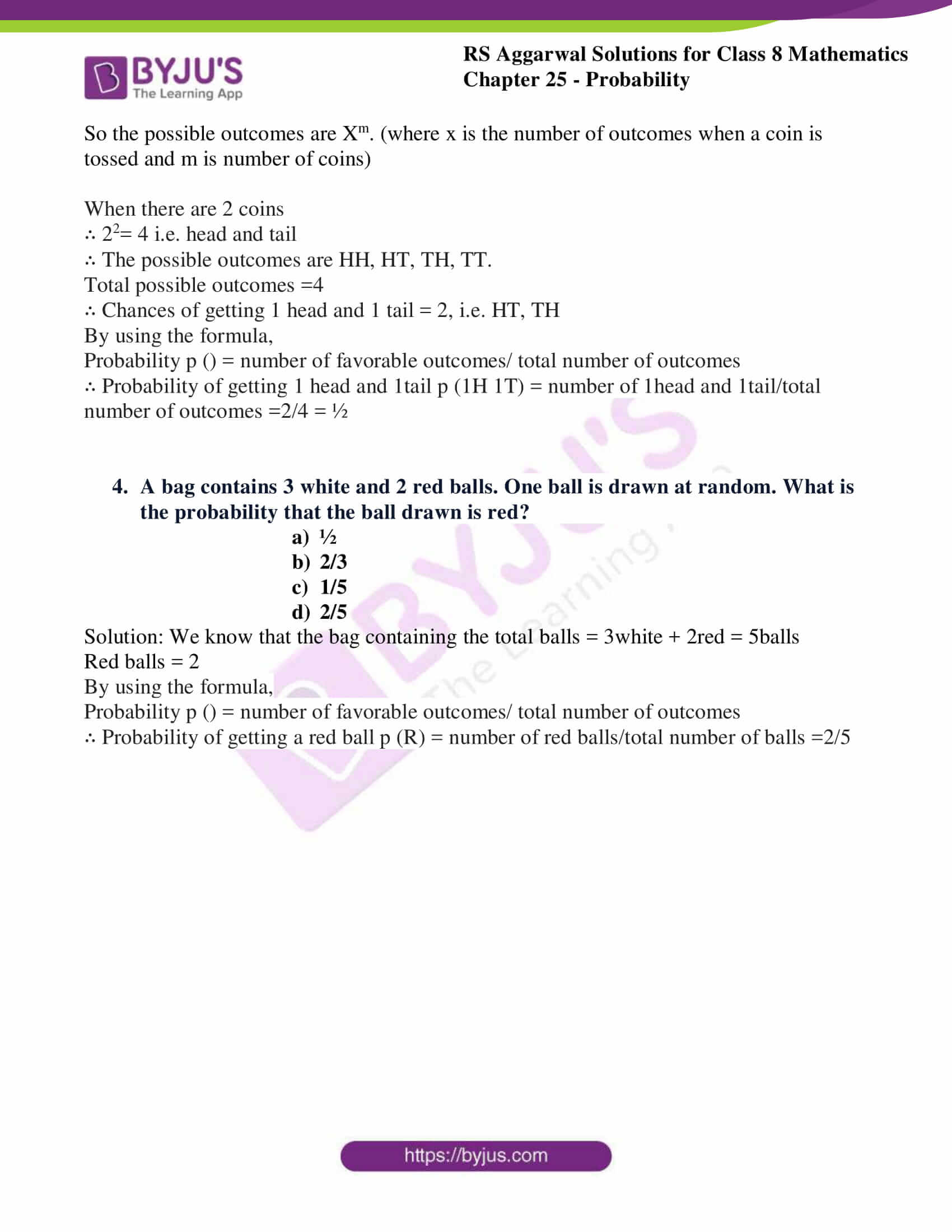## Access answers to RS Aggarwal Solutions for Class 8 Maths Chapter 25 – Probability

Exercise 25A
Q1.
(i) A coin is tossed. What are all possible outcomes?

(ii) Two coins are tossed simultaneously. What are all possible outcomes?

(iii) A die is thrown. What are all possible outcomes?

(iv) From a well-shuffled deck of 52 cards, one card is drawn at random. What is the number of all possible outcomes?

Solution: We know that the coin has two sides head (H) and tail (T)

So the possible outcomes are Xm. (where x is the number of outcomes when a coin is tossed and m is number of coins)

(i) ∴ 21= 2 i.e. head and tail

∴ The possible outcomes are H and T.

(ii) When there are 2 coins

∴ 22= 4 i.e. head and tail

∴ The possible outcomes are HH, HT, TH, TT.

(iii) We know that the die has 6 facesSo, they are 1, 2, and 3,4,5,6

∴ The possible outcomes are 1, 2, 3,4,5,6.

(iv) We know that the deck of cards has a total of 52 cardsSo, they are 52cards

∴ The possible outcomes are 52cards.

2. In a single throw of coin, what is the probability of getting a tail?
Solution:
We know that the coin has two sides head (H) and tail (T)
So the possible outcomes are Xm. (where x is the number of outcomes when a coin is tossed and m is number of coins)
∴ The possible outcomes are H and T.
Total possible outcomes =2
∴ Chances of getting a tail = 1(since there is a single coin)
By using the formula,
Probability (p) = number of favorable outcomes/ total number of outcomes
∴ Probability of getting a tail p (T) = chances/total number of outcomes = ½

3. In a single throw of two coins, find the probability of getting (i) both tails, (ii) at least 1 tail, (iii) at the most 1 tail.
Solution: We know that the coin has two sides head (H) and tail (T)
So the possible outcomes are Xm. (where x is the number of outcomes when a coin is tossed and m is number of coins)
(i) When there are 2 coins∴ 22= 4 i.e. head and tail
∴ The possible outcomes are HH, HT, TH, TT.
Total possible outcomes =4
∴ Chances of getting a 2 tails = 1, i.e. TT
By using the formula,
Probability (p) = number of favorable outcomes/ total number of outcomes
∴ Probability of getting a tail p (bothT) = number of two tails occurred/total number of outcomes = ¼
(ii) When there are 2 coins∴ 22= 4 i.e. head and tail
∴ The possible outcomes are HH, HT, TH, TT.
Total possible outcomes =4
∴ Chances of getting atleast 1 tail = 3, i.e. HT, TH, TT
By using the formula,
Probability (p) = number of favorable outcomes/ total number of outcomes
∴ Probability of getting a tail p (atleast 1T) = number of times 1 tail occurred/total number of outcomes =3/4
(iii) When there are 2 coins∴ 22= 4 i.e. head and tail
∴ The possible outcomes are HH, HT, TH, TT.
Total possible outcomes =4
∴ Chances of getting atmost 1 tail = 3, i.e. HT, TH, TT
By using the formula,
Probability (p) = number of favorable outcomes/ total number of outcomes
∴ Probability of getting a tail p (atmost 1T) = number of times 1 tail occurred/total number of outcomes =3/4

4. A bag contains 4 white and 5 blue balls. They are mixed thoroughly and one ball is drawn at random. What is the probability of getting (i) a white ball? (ii) A blue ball?Solution:
(i) We know that the bag containing the total balls = 4white + 5blue = 9ballsWhite balls = 4
By using the formula,
Probability (p) = number of favorable outcomes/ total number of outcomes
∴ Probability of getting a white ball p (W) = number of white balls/total number of balls =4/9
(ii) We know that the bag containing the total balls = 4white + 5blue = 9ballsBlue balls = 5
By using the formula,
Probability (p) = number of favorable outcomes/ total number of outcomes
∴ Probability of getting a blue ball p (B) = number of blue balls/total number of balls =5/9

5. A bag contains 5 white, 6 red and 4 green balls. One ball is drawn at random. What is the probability that the ball drawn is (i) green? (ii) White? (iii) Non-red?Solution:
(i) We know that the bag containing the total balls = 5white+6red+4green = 15ballsGreen balls = 4
By using the formula,
Probability (p) = number of favorable outcomes/ total number of outcomes
∴ Probability of getting a green ball p (G) = number of green balls/total number of balls =4/15
(ii) We know that the bag containing the total balls = 5white +6red+4green = 15ballsWhite balls = 5
By using the formula,
Probability (p) = number of favorable outcomes/ total number of outcomes
∴ Probability of getting a white ball p (W) = number of white balls/total number of balls =5/15=1/3
(iii) We know that the bag containing the total balls = 4white +6red+4green = 15ballsNumber of outcomes (excluding red) = 5white+4green=9balls
By using the formula,
Probability (p) = number of favorable outcomes/ total number of outcomes
∴ Probability of getting a green ball p (G) = number of white balls/total number of balls =9/15=3/5

6. In a lottery, there are 10 prizes and 20 blanks. A ticket is chosen at random. What is the probability of getting a prize? Solution: we know that the total number
of lottery tickets = 30
Number of lottery tickets having prize = 10
By using the formula,
Probability (p) = number of favorable outcomes/ total number of outcomes
∴ Probability of getting a prized ticket p(P) = number of prized tickets/total number of lottery tickets =10/30 =1/3

Exercise 25B

OBJECTIVE QUESTIONS

Select the correct answer in each of the following:

1. In a spinning wheel, there are 3 white and 5 green sectors. It is spinned. What is the probability of getting a green sector?

1. 5/3
2. 5/8
3. 1/5
4. 3/8

Solution: we know that the,
Total number of sectors= 3white+5green =8sectors
By using the formula,
Probability p () = number of favorable outcomes/ total number of outcomes
∴ Probability of getting a green sector p (G) = number of green sectors/total number of sectors =5/8

2. 8 cards are numbered as 1, 2, 3, 4, 5, 6, 7, 8 respectively. They are kept in a box and mixed thoroughly. Once card is chosen at random. What is the probability of getting a number less than
4?

1. ½
2. ¾
3. 3/8
4. 3/5

Solution: we know that the,
Total number of cards kept in the box = 8
∴ number of cards having a number less than 4 on it = 3
By using the formula,
Probability p () = number of favorable outcomes/ total number of outcomes
∴ Probability of selecting a card with a number less than 4 on it p(no.of cards less than 4) = number of cards having a number less than 4/total number of cards =3/8

3. Two coins are tossed simultaneously. What is the probability of getting one head and one tail?

1. ¼
2. ½
3. ¾
4. 2/3

Solution: We know that the coin has two sides head (H) and tail (T)
So the possible outcomes are Xm. (where x is the number of outcomes when a coin is tossed and m is number of coins)
When there are 2 coins
∴ 22= 4 i.e. head and tail
∴ The possible outcomes are HH, HT, TH, TT.
Total possible outcomes =4
∴ Chances of getting 1 head and 1 tail = 2, i.e. HT, TH
By using the formula,
Probability p () = number of favorable outcomes/ total number of outcomes
∴ Probability of getting 1 head and 1tail p (1H 1T) = number of 1head and 1tail/total number of outcomes =2/4 = ½

4. A bag contains 3 white and 2 red balls. One ball is drawn at random. What is the probability that the ball drawn is red?

1. ½
2. 2/3
3. 1/5
4. 2/5

Solution: We know that the bag containing the total balls = 3white + 2red = 5balls
Red balls = 2
By using the formula,
Probability p () = number of favorable outcomes/ total number of outcomes
∴ Probability of getting a red ball p (R) = number of red balls/total number of balls =2/5

## RS Aggarwal Solutions for Class 8 Maths Chapter 25 – Probability

Chapter 25 – Probability contains 2 exercises and the RS Aggarwal solutions present on this page provide the solutions for the questions present in each exercise. Now, let us have a look at the
concepts discussed in this chapter.

Probability of occurrence of an event.

### Chapter Brief of RS Aggarwal Solutions for Class 8 Maths Chapter 25 – Probability

In Chapter 25 – Probability of RS Aggarwal Solutions for Class 8 Maths, solutions are provided step-wise which makes it easier to understand the difficult problems.
As the Chapter is about Probability, in our daily life we use probability to make decisions when we don’t know exactly what the output would be. Most of the time we won’t perform actual probability but
instead, we use subjective probability to make judgemental calls and determine the best course of action. In the RS Aggarwal Solutions book, many such exercise problems are given goahead and solve them.